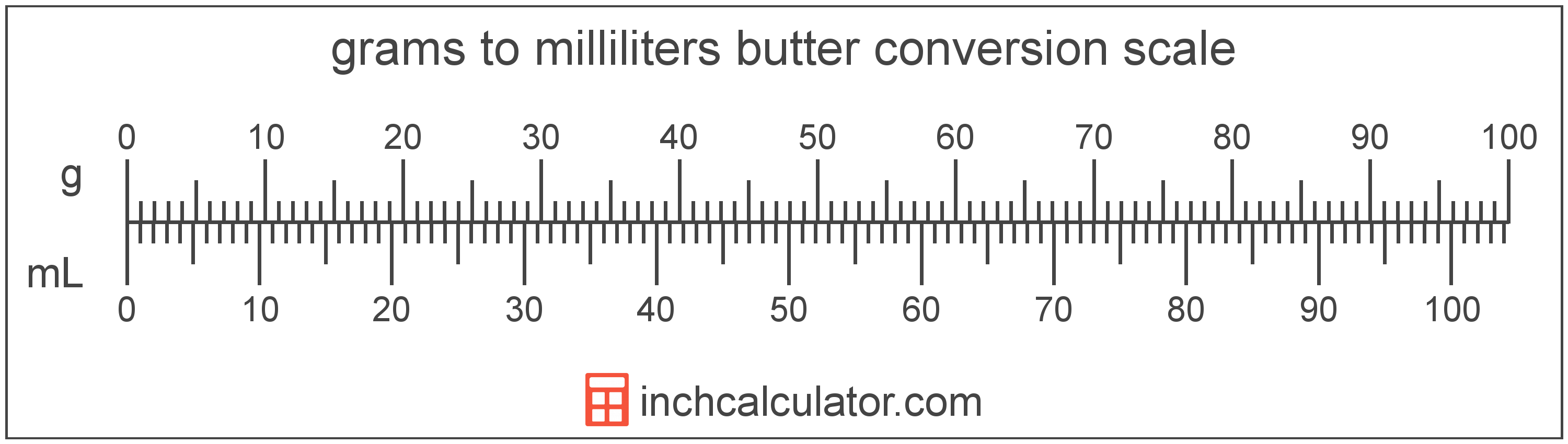# Convert Milliliters of Butter to Grams

Enter the butter in milliliters below to get the value converted to grams.

Results in Grams:1 mL = 0.958614 g

Do you want to convert grams to milliliters?

## How to Convert Milliliters to Grams

To convert a measurement in milliliters to a measurement in grams, multiply the butter by the following conversion ratio: 0.958614 grams/milliliter.

Since one milliliter of butter is equal to 0.958614 grams, you can use this simple formula to convert:

grams = milliliters × 0.958614

The butter in grams is equal to the butter in milliliters multiplied by 0.958614.

For example, here's how to convert 5 milliliters to grams using the formula above.
grams = (5 mL × 0.958614) = 4.79307 g### How Many Grams Are in a Milliliter of Butter?

There are 0.958614 grams in a milliliter of butter, which is why we use this value in the formula above.

1 mL = 0.958614 g

## What Is a Milliliter?

Butter is sometimes measured by volume in milliliters. One stick of butter contains about 118.3 milliliters.

The milliliter is an SI unit of volume in the metric system. A milliliter is sometimes also referred to as a millilitre. Milliliters can be abbreviated as mL; for example, 1 milliliter can be written as 1 mL.

## What Is a Gram?

Butter has a density of 911 grams per liter, and one stick of butter weighs about 113.4 grams.

The gram, or gramme, is an SI unit of weight in the metric system. Grams can be abbreviated as g; for example, 1 gram can be written as 1 g.

## Milliliter to Gram Conversion Table

Table showing various milliliter measurements converted to grams.
Milliliters Grams
1 mL 0.958614 g
2 mL 1.9172 g
3 mL 2.8758 g
4 mL 3.8345 g
5 mL 4.7931 g
6 mL 5.7517 g
7 mL 6.7103 g
8 mL 7.6689 g
9 mL 8.6275 g
10 mL 9.5861 g
11 mL 10.54 g
12 mL 11.5 g
13 mL 12.46 g
14 mL 13.42 g
15 mL 14.38 g
16 mL 15.34 g
17 mL 16.3 g
18 mL 17.26 g
19 mL 18.21 g
20 mL 19.17 g
21 mL 20.13 g
22 mL 21.09 g
23 mL 22.05 g
24 mL 23.01 g
25 mL 23.97 g
26 mL 24.92 g
27 mL 25.88 g
28 mL 26.84 g
29 mL 27.8 g
30 mL 28.76 g
31 mL 29.72 g
32 mL 30.68 g
33 mL 31.63 g
34 mL 32.59 g
35 mL 33.55 g
36 mL 34.51 g
37 mL 35.47 g
38 mL 36.43 g
39 mL 37.39 g
40 mL 38.34 g